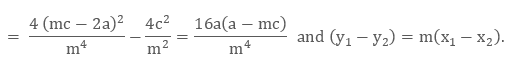×#### Thank you for registering.

One of our academic counsellors will contact you within 1 working day.

Click to Chat

1800-1023-196

+91-120-4616500

CART 0

• 0

MY CART (5)

Use Coupon: CART20 and get 20% off on all online Study Material

ITEM
DETAILS
MRP
DISCOUNT
FINAL PRICE
Total Price: Rs.

There are no items in this cart.
Continue Shopping```Chord of a ParabolaIntersection of a Straight Line with a Parabola

The combined equation of straight line y = mx + c and parabola

y2 = 4ax gives us the co-ordinates of point(s) of their intersection. The combined equation m2x2 + 2x (mc – 2a) + c2 = 0 will give those roots. The straight line therefore meets the parabola at two points.
Points of Intersection of a straight line with the parabola y2 = 4ax
Points of intersection of y2 = 4ax and y = mx + c are given by (mx+c)2=4ax
i.e. m2x2 + 2x(mc – 2a) + c2 = 0. …… (i)
Since (i) is a quadratic equation, the straight line meets the parabola in two points, real, coincident, or imaginary. The roots of (i) are real or imaginary according as {2(mx – 2a)}2 – 4m2c2 is positive or negative, i.e. according as – amc + a2 is positive or negative, i.e. according as mc is less than or greater than a, (taking a as positive).

Note:
When m is very small, one of the roots of equation (i) is very large; when m is equal to zero, this root is infinitely large. Hence every straight line parallel to the axis of the parabola meets the curve in one point at a finite distance and in another point at an infinite distance from the vertex. It means that a line parallel to the axis of theparabola meets the parabola only in one point.

Length of the chord

As in the preceding article, the abscissae of the points common to the straight line y = mx + c and the parabola y2 = 4ax are given by the equation m2x2 + (2mx – 4a) x + c2 = 0.Hence, the required length of chordllustration:
Find the Length of the chord intercepted by the parabola y2 = 4ax from the line y = mx + c. Also find its mid-point. Solution:
Simply by applying the formula o length of the joining (x1, y1) and (x2, y2) we get,
Length of the chord = √((x1-x2 )2+(y1-y2 )2 )
= √((x1-x2 )2+m2 (x1-x2 )2 )
= |x1 – x2| √(1+m2 ) = 4 √(a(a-mc) ) √(1+m2 )
[ ? x1+x2=(-2(m-2a) )/m2 and x1 x2=c2/m2 ]
The midpoint of the chord is ((2a-mc)/m2 ,2a/m)

To read more, Buy study materials of Parabola comprising study notes, revision notes, video lectures, previous year solved questions etc. Also browse for more study materials on Mathematics here.
```### Course Features

• 731 Video Lectures
• Revision Notes
• Previous Year Papers
• Mind Map
• Study Planner
• NCERT Solutions
• Discussion Forum
• Test paper with Video Solution